Anzeige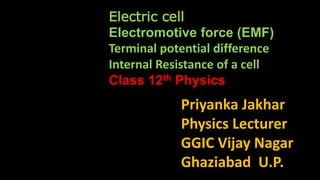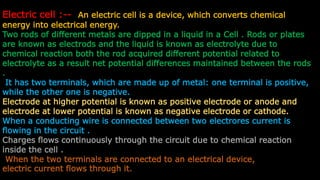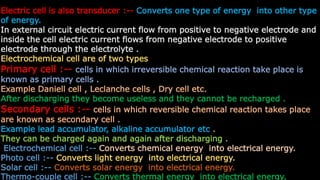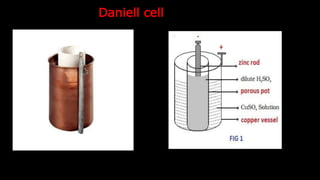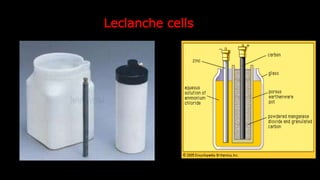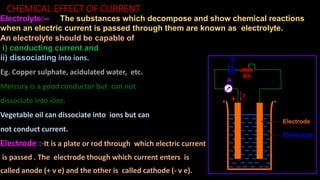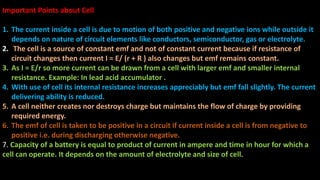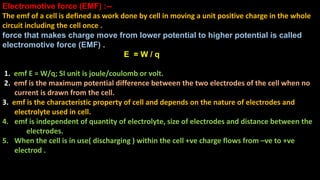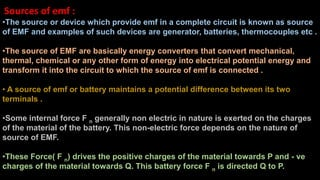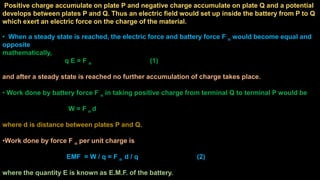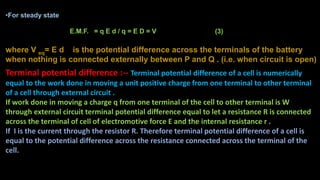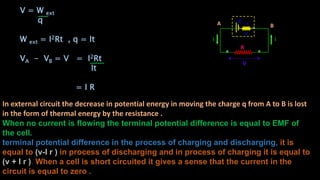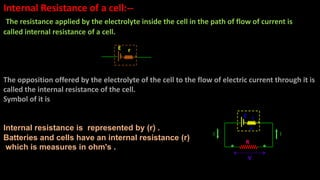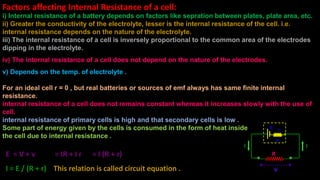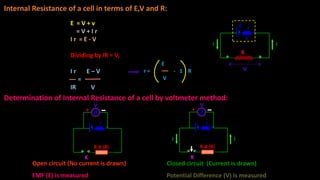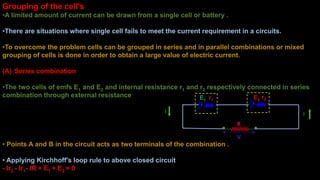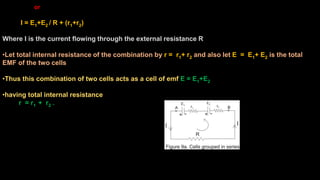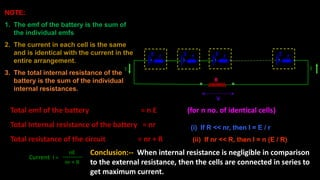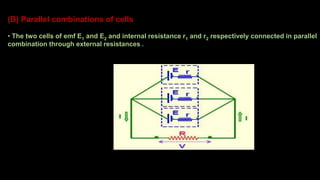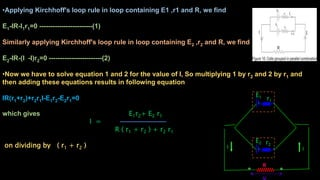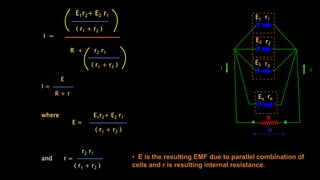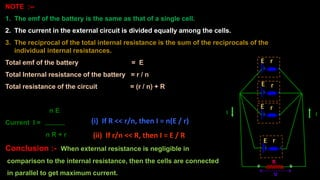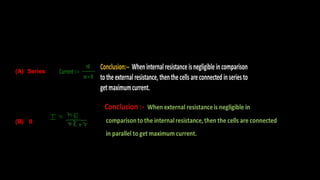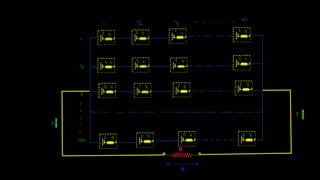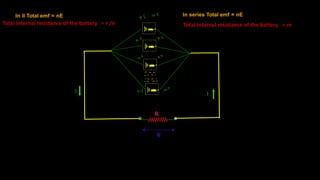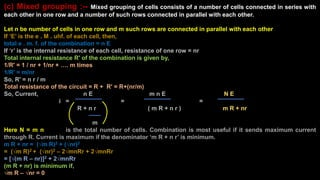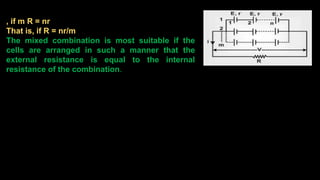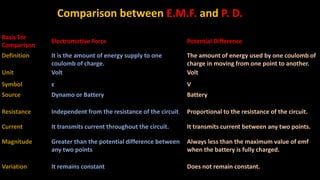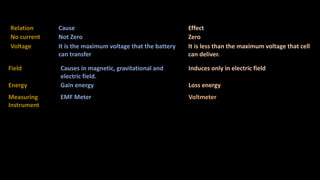1 von 29
Anzeige

### cell.ppt

1. Priyanka Jakhar Physics Lecturer GGIC Vijay Nagar Ghaziabad U.P. Electric cell Electromotive force (EMF) Terminal potential difference Internal Resistance of a cell Class 12th Physics
2. Electric cell :-- An electric cell is a device, which converts chemical energy into electrical energy. Two rods of different metals are dipped in a liquid in a Cell . Rods or plates are known as electrods and the liquid is known as electrolyte due to chemical reaction both the rod acquired different potential related to electrolyte as a result net potential differences maintained between the rods . It has two terminals, which are made up of metal: one terminal is positive, while the other one is negative. Electrode at higher potential is known as positive electrode or anode and electrode at lower potential is known as negative electrode or cathode. When a conducting wire is connected between two electrores current is flowing in the circuit . Charges flows continuously through the circuit due to chemical reaction inside the cell . When the two terminals are connected to an electrical device, electric current flows through it.
3. Electric cell is also transducer :-- Converts one type of energy into other type of energy. In external circuit electric current flow from positive to negative electrode and inside the cell electric current flows from negative electrode to positive electrode through the electrolyte . Electrochemical cell are of two types Primary cell :-- cells in which irreversible chemical reaction take place is known as primary cells . Example Daniell cell , Leclanche cells , Dry cell etc. After discharging they become useless and they cannot be recharged . Secondary cells :-- cells in which reversible chemical reaction takes place are known as secondary cell . Example lead accumulator, alkaline accumulator etc . They can be charged again and again after discharging . Electrochemical cell :-- Converts chemical energy into electrical energy. Photo cell :-- Converts light energy into electrical energy. Solar cell :-- Converts solar energy into electrical energy. Thermo-couple cell :-- Converts thermal energy into electrical energy.
4. Daniell cell
5. Leclanche cells
6. CHEMICAL EFFECT OF CURRENT Electrolyte:-- The substances which decompose and show chemical reactions when an electric current is passed through them are known as electrolyte. An electrolyte should be capable of i) conducting current and ii) dissociating into ions. Eg. Copper sulphate, acidulated water, etc. Mercury is a good conductor but can not dissociate into ions. Vegetable oil can dissociate into ions but can not conduct current. Electrode :-It is a plate or rod through which electric current is passed . The electrode though which current enters is called anode (+ v e) and the other is called cathode (- v e). E A I Rh Electrode Electrolyte
7. Important Points about Cell 1. The current inside a cell is due to motion of both positive and negative ions while outside it depends on nature of circuit elements like conductors, semiconductor, gas or electrolyte. 2. The cell is a source of constant emf and not of constant current because if resistance of circuit changes then current I = E/ (r + R ) also changes but emf remains constant. 3. As I = E/r so more current can be drawn from a cell with larger emf and smaller internal resistance. Example: In lead acid accumulator . 4. With use of cell its internal resistance increases appreciably but emf fall slightly. The current delivering ability is reduced. 5. A cell neither creates nor destroys charge but maintains the flow of charge by providing required energy. 6. The emf of cell is taken to be positive in a circuit if current inside a cell is from negative to positive i.e. during discharging otherwise negative. 7. Capacity of a battery is equal to product of current in ampere and time in hour for which a cell can operate. It depends on the amount of electrolyte and size of cell.
8. Electromotive force (EMF) :-- The emf of a cell is defined as work done by cell in moving a unit positive charge in the whole circuit including the cell once . force that makes charge move from lower potential to higher potential is called electromotive force (EMF) . E = W / q 1. emf E = W/q; SI unit is joule/coulomb or volt. 2. emf is the maximum potential difference between the two electrodes of the cell when no current is drawn from the cell. 3. emf is the characteristic property of cell and depends on the nature of electrodes and electrolyte used in cell. 4. emf is independent of quantity of electrolyte, size of electrodes and distance between the electrodes. 5. When the cell is in use( discharging ) within the cell +ve charge flows from –ve to +ve electrod .
9. Sources of emf : •The source or device which provide emf in a complete circuit is known as source of EMF and examples of such devices are generator, batteries, thermocouples etc . •The source of EMF are basically energy converters that convert mechanical, thermal, chemical or any other form of energy into electrical potential energy and transform it into the circuit to which the source of emf is connected . • A source of emf or battery maintains a potential difference between its two terminals . •Some internal force F n generally non electric in nature is exerted on the charges of the material of the battery. This non-electric force depends on the nature of source of EMF. •These Force( F n) drives the positive charges of the material towards P and - ve charges of the material towards Q. This battery force F n is directed Q to P.
10. Positive charge accumulate on plate P and negative charge accumulate on plate Q and a potential develops between plates P and Q. Thus an electric field would set up inside the battery from P to Q which exert an electric force on the charge of the material. • When a steady state is reached, the electric force and battery force F n would become equal and opposite mathematically, q E = F n (1) and after a steady state is reached no further accumulation of charge takes place. • Work done by battery force F n in taking positive charge from terminal Q to terminal P would be W = F n d where d is distance between plates P and Q. •Work done by force F n per unit charge is EMF = W / q = F n d / q (2) where the quantity E is known as E.M.F. of the battery.
11. •For steady state E.M.F. = q E d / q = E D = V (3) where V eq= E d is the potential difference across the terminals of the battery when nothing is connected externally between P and Q . (i.e. when circuit is open) Terminal potential difference :-- Terminal potential difference of a cell is numerically equal to the work done in moving a unit positive charge from one terminal to other terminal of a cell through external circuit . If work done in moving a charge q from one terminal of the cell to other terminal is W through external circuit terminal potential difference equal to let a resistance R is connected across the terminal of cell of electromotive force E and the internal resistance r . If I is the current through the resistor R. Therefore terminal potential difference of a cell is equal to the potential difference across the resistance connected across the terminal of the cell.
12. In external circuit the decrease in potential energy in moving the charge q from A to B is lost in the form of thermal energy by the resistance . When no current is flowing the terminal potential difference is equal to EMF of the cell. terminal potential difference in the process of charging and discharging, it is equal to (v-I r ) in process of discharging and in process of charging it is equal to (v + I r ). When a cell is short circuited it gives a sense that the current in the circuit is equal to zero . R rE II V v V = W ext q W ext = I2Rt , q = It VA - VB = V = I2Rt It = I R A B
13. Internal Resistance of a cell:-- The resistance applied by the electrolyte inside the cell in the path of flow of current is called internal resistance of a cell. The opposition offered by the electrolyte of the cell to the flow of electric current through it is called the internal resistance of the cell. Symbol of it is Internal resistance is represented by (r) . Batteries and cells have an internal resistance (r) which is measures in ohm's . R rE II V v E r
14. Factors affecting Internal Resistance of a cell: i) Internal resistance of a battery depends on factors like sepration between plates, plate area, etc. ii) Greater the conductivity of the electrolyte, lesser is the internal resistance of the cell. i.e. internal resistance depends on the nature of the electrolyte. iii) The internal resistance of a cell is inversely proportional to the common area of the electrodes dipping in the electrolyte. iv) The internal resistance of a cell does not depend on the nature of the electrodes. v) Depends on the temp. of electrolyte . For an ideal cell r = 0 , but real batteries or sources of emf always has same finite internal resistance. internal resistance of a cell does not remains constant whereas it increases slowly with the use of cell. internal resistance of primary cells is high and that secondary cells is low . Some part of energy given by the cells is consumed in the form of heat inside the cell due to internal resistance . R rE II V v E = V + v = IR + I r = I (R + r) I = E / (R + r) This relation is called circuit equation .
15. Internal Resistance of a cell in terms of E,V and R: R rE II V v E = V + v = V + I r I r = E - V Dividing by IR = V, I r E – V = IR V Determination of Internal Resistance of a cell by voltmeter method: r K R.B (R) V + r II R.B (R) K V + Open circuit (No current is drawn) EMF (E) is measured Closed circuit (Current is drawn) Potential Difference (V) is measured E r = - 1 R V
16. Grouping of the cell's •A limited amount of current can be drawn from a single cell or battery . •There are situations where single cell fails to meet the current requirement in a circuits. •To overcome the problem cells can be grouped in series and in parallel combinations or mixed grouping of cells is done in order to obtain a large value of electric current. (A) Series combination •The two cells of emfs E1 and E2 and internal resistance r1 and r2 respectively connected in series combination through external resistance • Points A and B in the circuit acts as two terminals of the combination . • Applying Kirchhoff's loop rule to above closed circuit - Ir2 - Ir1- IR + E1 + E2 = 0 R I V r1E1 r2E2 I
17. or I = E1+E2 / R + (r1+r2) Where I is the current flowing through the external resistance R •Let total internal resistance of the combination by r = r1+ r2 and also let E = E1+ E2 is the total EMF of the two cells •Thus this combination of two cells acts as a cell of emf E = E1+E2 •having total internal resistance r = r1 + r2 .
18. rE rErE rE R NOTE: 1. The emf of the battery is the sum of the individual emfs 2. The current in each cell is the same and is identical with the current in the entire arrangement. 3. The total internal resistance of the battery is the sum of the individual internal resistances. I Total emf of the battery = n E (for n no. of identical cells) Total Internal resistance of the battery = nr Total resistance of the circuit = nr + R (i) If R << nr, then I = E / r (ii) If nr << R, then I = n (E / R) Current I = nE nr + R Conclusion:-- When internal resistance is negligible in comparison to the external resistance, then the cells are connected in series to get maximum current. I V
19. (B) Parallel combinations of cells • The two cells of emf E1 and E2 and internal resistance r1 and r2 respectively connected in parallel combination through external resistances .
20. •Applying Kirchhoff's loop rule in loop containing E1 ,r1 and R, we find E1-IR-I1r1=0 ------------------------(1) Similarly applying Kirchhoff's loop rule in loop containing E2 ,r2 and R, we find E2-IR-(I -I)r2=0 ------------------------(2) •Now we have to solve equation 1 and 2 for the value of I, So multiplying 1 by r2 and 2 by r1 and then adding these equations results in following equation IR(r1+r2)+r2r1I-E1r2-E2r1=0 which gives E1r2+ E2 r1 I = R ( r1 + r2 ) + r2 r1 on dividing by ( r1 + r2 ) R II r1 E1 r2 E2
21. E1r2+ E2 r1 ( r1 + r2 ) I = R + r2 r1 ( r1 + r2 ) E I = R + r where E1r2+ E2 r1 E = ( r1 + r2 ) r2 r1 and r = ( r1 + r2 ) • E is the resulting EMF due to parallel combination of cells and r is resulting internal resistance. V II E2 E1 E3 En rn r1 r2 r3 R
22. NOTE :-- 1. The emf of the battery is the same as that of a single cell. 2. The current in the external circuit is divided equally among the cells. 3. The reciprocal of the total internal resistance is the sum of the reciprocals of the individual internal resistances. Total emf of the battery = E Total Internal resistance of the battery = r / n Total resistance of the circuit = (r / n) + R n E Current I = n R + r Conclusion :- When external resistance is negligible in comparison to the internal resistance, then the cells are connected in parallel to get maximum current. (i) If R << r/n, then I = n(E / r) (ii) If r/n << R, then I = E / R V II E E E E r r r r R
23. (A) Series (B) II
24. V I I
25. In series Total emf = nE Total Internal resistance of the battery = nrTotal Internal resistance of the battery = r /n In II Total emf = nE V II
26. (c) Mixed grouping :-- Mixed grouping of cells consists of a number of cells connected in series with each other in one row and a number of such rows connected in parallel with each other. Let n be number of cells in one row and m such rows are connected in parallel with each other If ‘E’ is the e . M . uhf. of each cell, then, total e . m. f. of the combination = n E If ‘r’ is the internal resistance of each cell, resistance of one row = nr Total internal resistance R' of the combination is given by, 1/R' = 1 / nr + 1/nr + …. m times 1/R' = m/nr So, R' = n r / m Total resistance of the circuit = R + R' = R+(nr/m) So, Current, n E m n E N E i = = = R + n r ( m R + n r ) m R + nr m Here N = m n is the total number of cells. Combination is most useful if it sends maximum current through R. Current is maximum if the denominator ‘m R + n r’ is minimum. m R + nr = (√m R)2 + (√nr)2 = (√m R)2 + (√nr)2 – 2√mnRr + 2√mnRr = [√(m R – nr)]2 + 2√mnRr (m R + nr) is minimum if, √m R – √nr = 0
27. , if m R = nr That is, if R = nr/m The mixed combination is most suitable if the cells are arranged in such a manner that the external resistance is equal to the internal resistance of the combination.
28. Basis For Comparison Electromotive Force Potential Difference Definition It is the amount of energy supply to one coulomb of charge. The amount of energy used by one coulomb of charge in moving from one point to another. Unit Volt Volt Symbol ε V Source Dynamo or Battery Battery Resistance Independent from the resistance of the circuit Proportional to the resistance of the circuit. Current It transmits current throughout the circuit. It transmits current between any two points. Magnitude Greater than the potential difference between any two points Always less than the maximum value of emf when the battery is fully charged. Variation It remains constant Does not remain constant. Comparison between E.M.F. and P. D.
29. Field Causes in magnetic, gravitational and electric field. Induces only in electric field Energy Gain energy Loss energy Measuring Instrument EMF Meter Voltmeter Relation Cause Effect No current Not Zero Zero Voltage It is the maximum voltage that the battery can transfer It is less than the maximum voltage that cell can deliver.
Anzeige